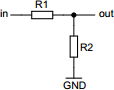# Resistive voltage divider

## Calculates properties of resistive voltage divider circuit

 Example 1: Must calculate the input voltage when output voltage is 500 millivolts using 120 kilo-ohm source and 10 kilo-ohm sink resistors View example Example 2: Must calculate the source resistor to have 1 volt output at 100 volt input using 2.2 kilo-ohm sink resistor View example
 Input voltage volts Output voltage volts Source resistor (R1) ohms Sink resistor (R2) ohmsYou may use one of the following SI prefix after a value: p=pico, n=nano, u=micro, m=milli, k=kilo, M=mega, G=giga

 Results Source resistor (R1) 217.8 kilo-ohms Voltage drop of source resistor (R1) 99 volts Power of source resistor (R1) 45 milliwatts Power of sink resistor (R2) ~454.545455 microwatts Total power of the circuit ~45.454545 milliwatts Total current of the circuit ~454.545455 microamperes